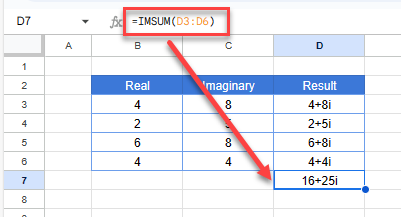# Do Math With Imaginary Numbers in Excel & Google Sheets

This tutorial demonstrates how to do math with imaginary numbers in Excel and Google Sheets.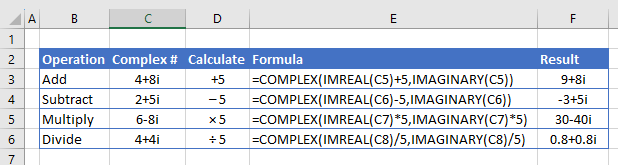## Real, Imaginary, and Complex Numbers

Real numbers are what we are all familiar with and use in everyday math. More complicated mathematical principles, such as differential equations, involve the use of complex numbers. Complex numbers combine real and imaginary numbers. Imaginary numbers are written as a real number multiplied by the imaginary unit i, where i is the square root of negative 1. Complex numbers are shown as a+bi, where a is the real part of the number, and b is the coefficient for the imaginary part of the number.

So for example, in the complex number 5+3i, 5 is the real part of the number, and 3i is the imaginary part.

### Express Complex Numbers in Excel

Consider the following table of complex numbers. Column B has the real (a) part of each number, and Column C is the coefficient (b) for the imaginary part.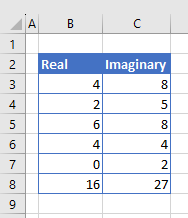1. Type the following formula in the column to the right of the first imaginary number to create a complex number.
`=COMPLEX(B3,C3)`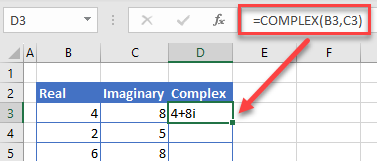1. If you wish to change the suffix from i to j, you can specify this by selecting it in the formula. As it is an optional argument, if you leave it blank, then i is assumed.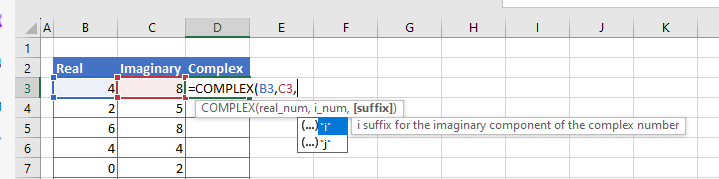1. Copy the formula down to populate the rest of the rows with the formula.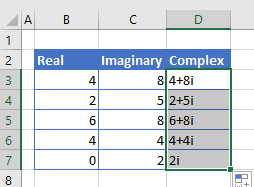### Extract Real and Imaginary Numbers From Complex Numbers

Starting with complex numbers, you can extract the real and imaginary parts with formulas. Consider the following data: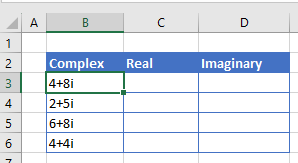To extract the real part of the complex number, use the IMREAL Function.

`=IMREAL(B3)`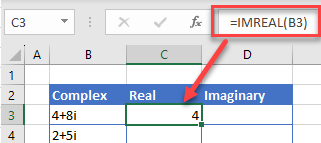To extract the imaginary part of the complex number, use the IMAGINARY Function.

`=IMAGINARY(B3)`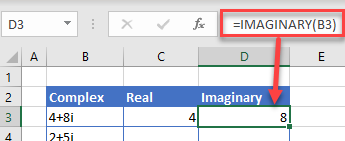## Math With Real and Imaginary Numbers

Complex numbers are stored as text in Excel, so you cannot add, subtract, multiply, and divide with complex numbers in the same way you would with ordinary rational (real) numbers.

If you try to add an ordinary number to a complex number with the standard Excel formula, you get a #VALUE error.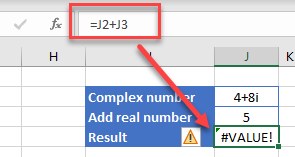To add 5 to the real part of the complex number, first extract the real part of the complex number. Then add 5 to the real part and recreate the complex number.

`=COMPLEX(IMREAL(J2)+J3,IMAGINARY(J2))`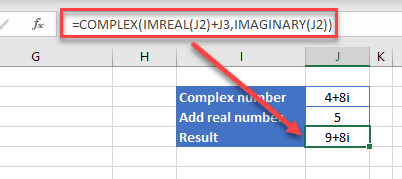For subtraction, replace the addition sign with the subtraction sign.

`=COMPLEX(IMREAL(J2)-J3,IMAGINARY(J2))`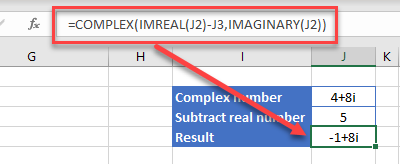The mathematical operation (addition or subtraction) is performed on the real number only and then a new complex number is created.

### Multiplication and Division

For multiplication, we use the multiply sign: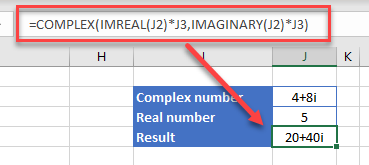=COMPLEX(IMREAL(J2)*J3,IMAGINARY(J2)*J3)

and for division, the division sign: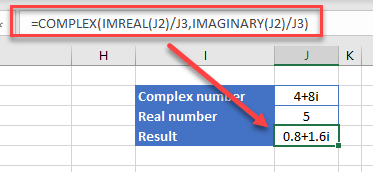=COMPLEX(IMREAL(J2)/J3,IMAGINARY(J2)/J3)

For multiplication and division, the mathematical operation is performed both on the real number and the coefficient of the imaginary number.

### Easy Excel Automation## Add Two Complex Numbers Together

The simplest way to add complex numbers together is to use the IMSUM Function.

`=IMSUM(D3:D7)`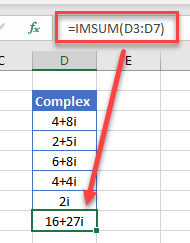You could, alternatively, add up the real column and add up the imaginary column, and then create a complex number sum from the totals of those columns.

1. Use the SUM Function to add up Columns B and C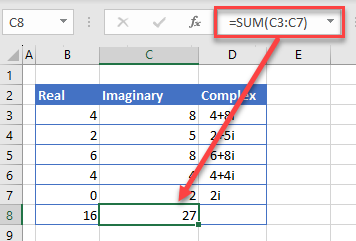1. Then use the COMPLEX function to get the total amount.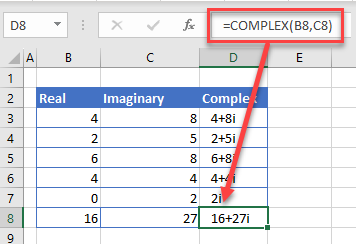This converts the 2 individual sum’s back to a COMPLEX number.  You will see that this is the same value as calculated using the IMSUM function.

## Math With Imaginary Numbers in Google Sheets

Google Sheets handles real, complex, and imaginary numbers just like Excel.

Use the COMPLEX Function to create a complex number.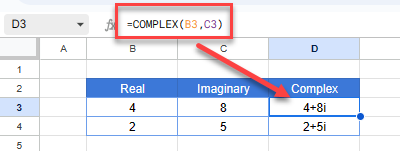Extract real and imaginary numbers from a complex number using the IMREAL and IMAGINARY Functions. Then add, subtract, multiply, or divide the same way you would in Excel.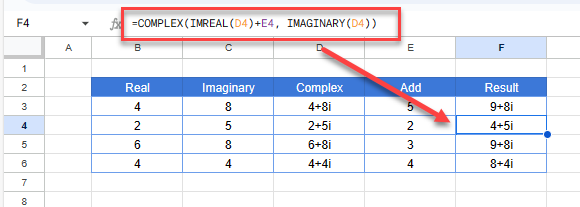Use the IMSUM Function to add several complex numbers.